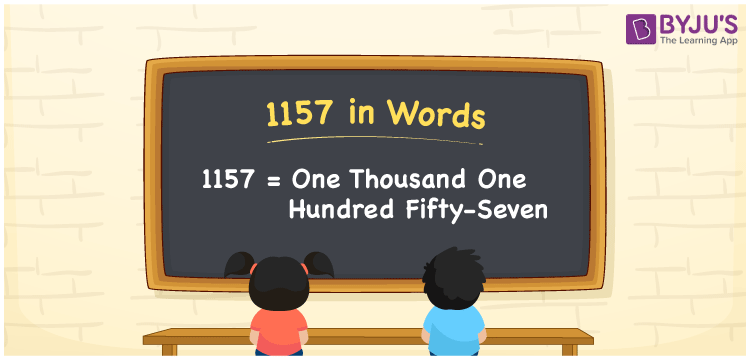# 1157 in words

1157 in words is written as One Thousand One Hundred and Fifty Seven. 1157 represents the count or value. The article on Counting Numbers can give you an idea about count or counting. The number 1157 is used in expressions that relate to money, distance, length, year and others. Let us consider an example for 1157. ”One Thousand One Hundred and Fifty Seven is the number of steps I took to reach my school”.

 1157 in words One Thousand One Hundred and Fifty Seven One Thousand One Hundred and Fifty Seven in Numbers 1157

## 1157 in English Words## How to Write 1157 in Words?

We can convert 1157 to words using a place value chart. The number 1157 has 4 digits, so let’s make a chart that shows the place value up to 4 digits.

 Thousands Hundreds Tens Ones 1 1 5 7

Thus, we can write the expanded form as:

1 × Thousand + 1 × Hundred + 5 × Ten + 7 × One

= 1 × 1000 + 1 × 100 + 5 × 10 + 7 × 1

= 1157

= One Thousand One Hundred and Fifty Seven.

1157 is the natural number that is succeeded by 1156 and preceded by 1158.

1157 in words – One Thousand One Hundred and Fifty Seven.

Is 1157 an odd number? – Yes.

Is 1157 an even number? – No.

Is 1157 a perfect square number? – No.

Is 1157 a perfect cube number? – No.

Is 1157 a prime number? – No.

Is 1157 a composite number? – Yes.

## Solved Example

1. Write the number 1157 in expanded form

Solution: 1 × 1000 + 1 × 100 + 5 × 10 + 7 × 1

We can write 1157 = 1000 +100 +50 + 7

= 1 × 1000 + 1 × 100 + 5 × 10 + 7 × 1

## Frequently Asked Questions on 1157 in words

Q1

### How to write 1157 in words?

1157 in words is written as One Thousand One Hundred and Fifty Seven.
Q2

### Is 1157 a perfect square number?

No. 1157 is not a perfect square number.
Q3

### Is 1157 a prime number?

No. 1157 is not a prime number.